# Benchmark: Process Costs under V8 using Google Apps Script

Gists

• March 22, 2020
• Published.

Kanshi Tanaike

# Introduction

V8 engine got to be able to be used at Google Apps Script. By this, I have reported about the process costs with and without using V8. Ref It is considered that knowing the process costs for various methods will be useful for creating the applications with Google Apps Script. Here, I would like to introduce the process costs of each situations under V8. The situations which measured the cost are as follows.

1. With and without Arrow function
2. “includes” and “indexOf”
3. With and without Destructuring Assignment
4. With and without Map object
5. “Array.prototype.push.apply”, Spread syntax and “concat”
6. only “reduce”, “Object.assign” and Spread syntax
7. “Array.from”, only “map” and “Object.entries”

# Experimental procedure

In this report, the following 2 dimensional array was used for the sample value. 1 dimensional array including 3 strings for searching was used as a source array. The 3 string values were put to the top, center and end of the array in order to measure the same condition. In this case, it uses all of elements in the array. The script for creating this sample array can be seen at here.

By the way, at GAS, the processing time is not stable as you know. So the average value for more than 250 times measurements was used for each data point which is shown by figures. At this time, the fluctuation of the average values was less than 1 %. I worry that each detailed-data point at my environment might be different from that at other user’s environment. But I think that the trend of this result can be used.

# Results and discussions

## 1. With and without Arrow function

The sample script is as follows. The sample script retrieves the rows which include searchText. In this measurement, the array (ar) of 3,000,000 rows and 10 columns was used.

const withArrowFunction = ar =>
ar.filter(row => row.some(col => col === searchText));

const withoutArrowFunction = ar =>
ar.filter(function(row) {
return row.some(function(col) {
return col === searchText;
});
});


The result is as follows.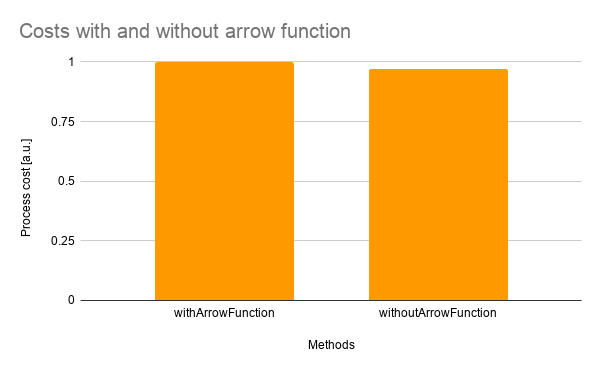From the result, it was found that the process cost with and without the arrow function was almost the same.

## 2. “includes” and “indexOf”

The sample script is as follows. The sample script retrieves the rows which include searchText. In this measurement, the array (ar) of 3,000,000 rows and 10 columns was used.

const withIncludes = ar => ar.filter(row => row.includes(searchText));

const withIndexOf = ar => ar.filter(row => row.indexOf(searchText) > -1);


The result is as follows.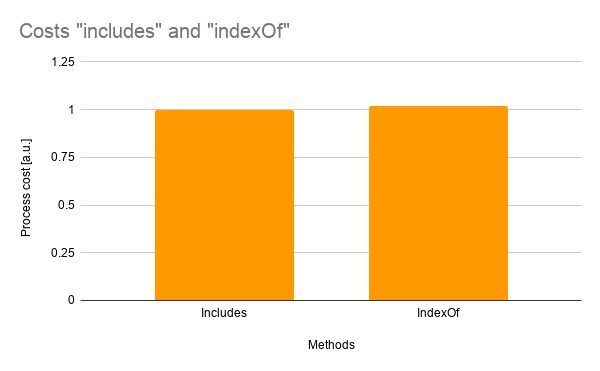From the result, it was found that the process cost “includes” and “indexOf” was almost the same.

## 3. With and without Destructuring Assignment

The sample script is as follows. The sample script retrieves the rows when the values of columns “A”, “E” and “J” are searchText. In this measurement, the array (ar) of 3,000,000 rows and 10 columns was used.

const withDestructuringAssignment = ar =>
ar.filter(
([a, , , , e, , , , , j]) =>
a === searchText || e === searchText || j === searchText
);

const withoutDestructuringAssignment = ar =>
ar.filter(
row =>
row === searchText || row === searchText || row === searchText
);


The result is as follows.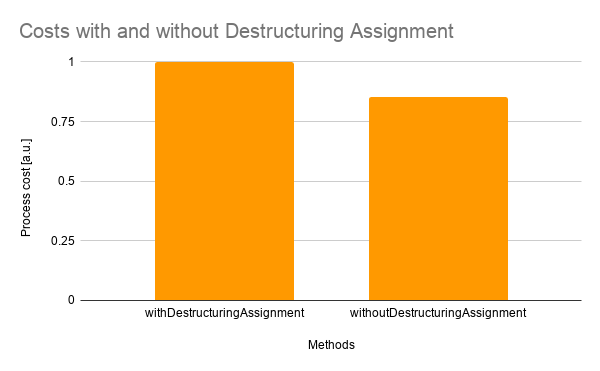From the result, it was found that when the destructuring assignment is used, the cost was about 15 % higher than that without the destructuring assignment.

## 4. With and without Map object

The sample script is as follows. The sample script removes the duplicated rows. In this measurement, the array (ar) of 3,000,000 rows and 10 columns was used.

const withMap = ar =>
Object.fromEntries(
ar.reduce((m, [a, , , , e, , , , , j]) => {
if (a === searchText || e === searchText || j === searchText)
m.set(searchText, m.has(searchText) ? m.get(searchText) + 1 : 1);
return m;
}, new Map())
);

const withoutMap = ar =>
ar.reduce((o, [a, , , , e, , , , , j]) => {
if (a === searchText || e === searchText || j === searchText)
o[searchText] = searchText in o ? o[searchText] + 1 : 1;
return o;
}, {});


The result is as follows.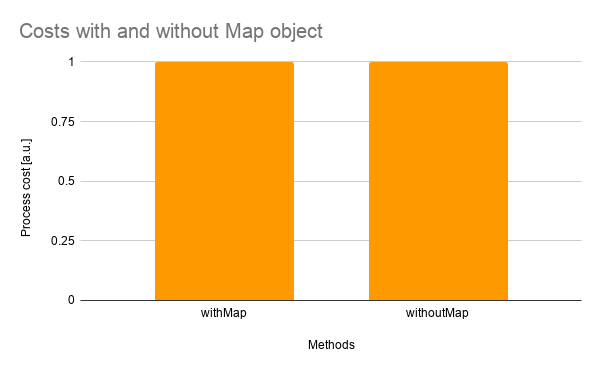From the result, it was found that the process cost with and without Map object was almost the same. But in this case, the cost of Object.fromEntries is added. It was found that when Object.fromEntries is not used, the cost with Map object was about 20 % lower than that without Map object.

## 5. “Array.prototype.push.apply”, Spread syntax and “concat”

The sample script is as follows. The sample script merges all rows. The costs of Array.prototype.push.apply, Spread syntax and concat were measured. In this measurement, the array (ar) of 3,000 rows and 10 columns was used.

const arrayPrototypePushApply = ar =>
ar.reduce((a, row) => {
Array.prototype.push.apply(a, row);
return a;
}, []);

const spreadSyntax = ar => ar.reduce((a, row) => [...a, row], []);

const concat = ar => ar.reduce((a, row) => a.concat(row), []);


The result is as follows.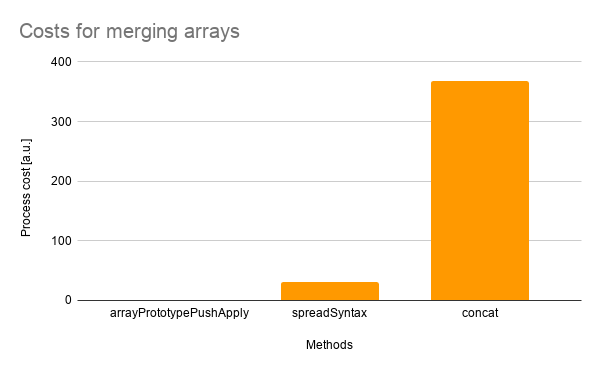From the result, it was found that the process cost of Array.prototype.push was the lowest of 3 methods. The costs of Spread syntax and concat were about 3,040 % and 36,666 % higher than that of Array.prototype.push, respectively.

### IMPORTANT POINT

When Array.prototype.push.apply is used, there is an important point. Please check “Limitation of Array.prototype.push.apply under V8 for Google Apps Script”.

## 6. only “reduce”, “Object.assign” and Spread syntax

The sample script is as follows. The sample script converts array to JSON object by removing duplicating. The costs of only reduce, Object.assign and the spread syntax were measured. In this measurement, the array (ar) of 3,000 rows and 10 columns was used.

const withoutSpreadSyntax = ar =>
ar.reduce((o, row) => {
if (row != searchText) o[row] = row;
return o;
}, {});

const withObjectAssign = ar =>
ar.reduce((o, row) => {
if (row != searchText) return Object.assign(o, { [row]: row });
return o;
}, {});

ar.reduce((o, row) => {
if (row != searchText) return { ...o, [row]: row };
return o;
}, {});


The result is as follows.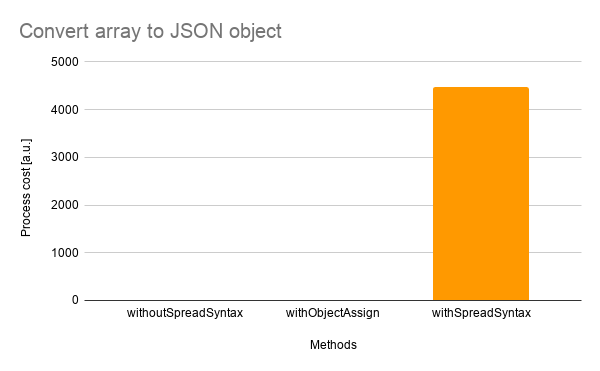From the result, it was found that the process cost of only reduce without the spread syntax and Object.assign was the lowest of 3 methods. The costs of Object.assign and Spread syntax were about 265 % and 448,063 % higher than that of only reduce, respectively.

## 7. “Array.from”, only “map” and “Object.entries”

The sample script is as follows. The sample script converts JSON object to array. The costs of Array.from, only map and Object.entries were measured. In this measurement, the array (ar) of 300,000 rows and 10 columns was used.

const withArrayFrom = obj => Array.from(Object.keys(obj), e => [e, obj[e]]);

const withoutObjectEntries = obj => Object.keys(obj).map(e => [e, obj[e]]);

const withObjectEntries = obj => Object.entries(obj);


The result is as follows.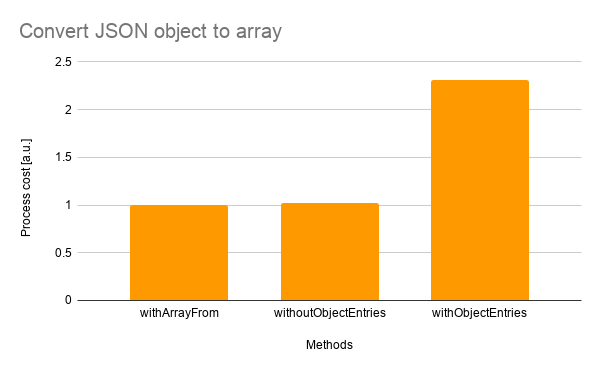From the result, it was found that the process costs of Array.from and only map were almost the same. The cost of Object.entries was about 131 % higher than that of Array.from.

# Summary

In this report, the process costs of 7 situations under V8 were measured. As the result, the following results could be obtained.

1. Process cost with and without the arrow function was almost the same.
2. Process cost “includes” and “indexOf” was almost the same.
3. When the destructuring assignment is used, the cost was about 15 % higher than that without the destructuring assignment.
4. Process cost with and without Map object was almost the same.
• But in this case, the cost of Object.fromEntries is added for retrieving the result as the object. And when Object.fromEntries is not used, the cost with Map object was about 20 % lower than that without Map object.
5. Process cost of Array.prototype.push was the lowest of Array.prototype.push.apply, Spread syntax and concat. The costs of Spread syntax and concat were about 3,040 % and 36,666 % higher than that of Array.prototype.push, respectively.
6. Process cost of only reduce was the lowest of only reduce, Object.assign and Spread syntax. The costs of Object.assign and Spread syntax were about 265 % and 448,063 % higher than that of only reduce, respectively.
7. Process costs of Array.from and only map were almost the same. The cost of Object.entries was about 131 % higher than that of Array.from.

# Note

• As a note, I have to describe that this is the result for Google Apps Script. For other languages, this result might be difference. And also, the process cost of this report might be modified by future update of Google.
• I think that there are various situations except for above situations. So I would like to measure more situations in the future.

Top